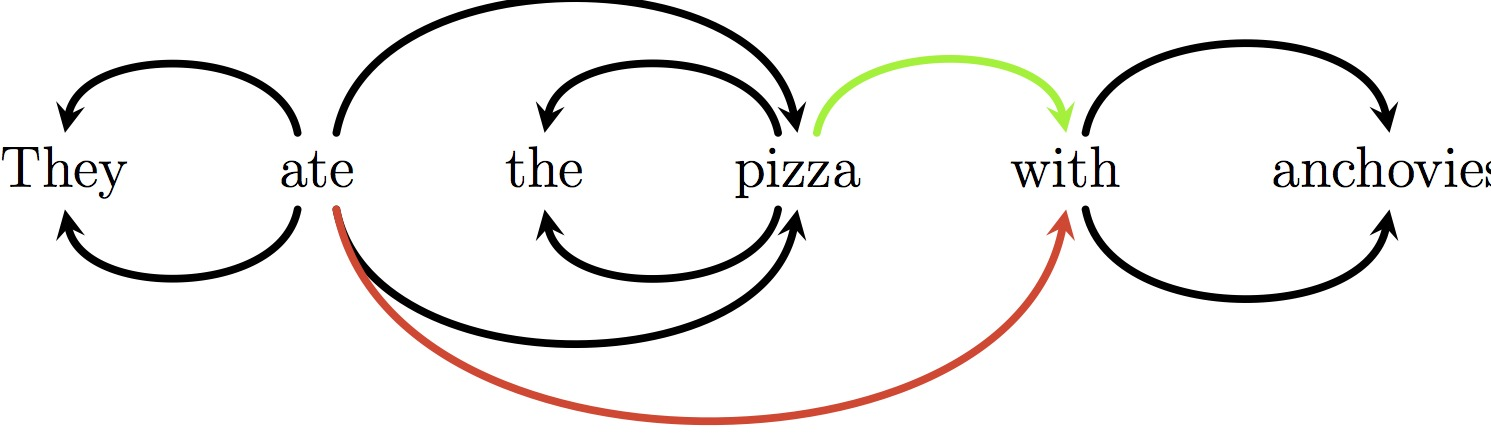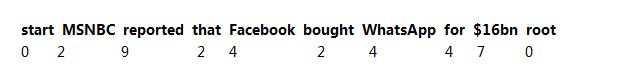### Python编程语言

Python 是一种面向对象、解释型计算机程序设计语言，由Guido van Rossum于1989年底发明，第一个公开发行版发行于1991年。Python语法简洁而清晰，具有丰富和强大的类库。它常被昵称为胶水语言，它能够把用其他语言制作的各种模块（尤其是C/C++）很轻松地联结在一起。Cython 系统和 Redshift 是为我目前的研究而写的。和麦考瑞大学的合同到期后，我计划六月份对它进行改进，用于一般用途。目前的版本托管在 GitHub 上。

Set volume to zero when I'm in a meeting, unless John's school calls.

Unless John's school calls, when I'm in a meeting, set volume to zero

Unless John's school, call when I'm in a meeting

``````>>> parser = parser.Parser()
>>> tokens = "Set the volume to zero when I 'm in a meeting unless John 's school calls".split()
[-1, 2, 0, 0, 3, 0, 7, 5, 7, 10, 8, 0, 13, 15, 15, 11]
>>> for i, h in enumerate(heads):
...  print(tokens[i] + ' <-- ' + head])
Set <-- None
the <-- volume
volume <-- Set
to <-- Set
zero <-- to
when <-- Set
I <-- 'm
'm <-- when
in <-- 'm
a <-- meeting
meeting <-- in
unless <-- Set
John <-- 's
's  <-- calls
school <-- calls
calls <-- unless``````

树。除了根外，每个单词都有一个弧头。
映射关系。针对每对依赖关系 （a1, a2）和 （b1, b2），如果 a1 < b2, 那么 a2 >= b2。换句话说，依赖关系不能交叉。不可能存在一对 a1 b1 a2 b2 或者 b1 a1 b2 a2 形式的依赖关系。``````class Parse(object):
def __init__(self, n):
self.n = n
self.lefts = []
self.rights = []
for i in range(n+1):
self.lefts.append(DefaultList(0))
self.rights.append(DefaultList(0))

else:

•     一个索引 i, 活动于符号列表中
•     到现在为止语法解析器中的加入的依赖关系
•     一个包含索引 i 之前产生的单词的栈，我们已为这些单词声明了弧头。

``````SHIFT = 0; RIGHT = 1; LEFT = 2
MOVES = [SHIFT, RIGHT, LEFT]

def transition(move, i, stack, parse):
global SHIFT, RIGHT, LEFT
if move == SHIFT:
stack.append(i)
return i + 1
elif move == RIGHT:
return i
elif move == LEFT:
return i
raise GrammarError("Unknown move: %d" % move)``````

LEFT 和 RIGHT 操作添加依赖关系并弹栈，而 SHIFT 压栈并增加缓存中 i 值。

``````class Parser(object):
...
def parse(self, words):
tags = self.tagger(words)
n = len(words)
idx = 1
stack = 
deps = Parse(n)
while stack or idx < n:
features = extract_features(words, tags, idx, n, stack, deps)
scores = self.model.score(features)
valid_moves = get_valid_moves(i, n, len(stack))
next_move = max(valid_moves, key=lambda move: scores[move])
idx = transition(next_move, idx, stack, parse)
return tags, parse

def get_valid_moves(i, n, stack_depth):
moves = []
if i < n:
moves.append(SHIFT)
if stack_depth >= 2:
moves.append(RIGHT)
if stack_depth >= 1:
moves.append(LEFT)
return moves``````

``````class Perceptron(object)
...
def score(self, features):
all_weights = self.weights
scores = dict((clas, 0) for clas in self.classes)
for feat, value in features.items():
if value == 0:
continue
if feat not in all_weights:
continue
weights = all_weights[feat]
for clas, weight in weights.items():
scores[clas] += value * weight
return scores``````

•     缓存中的前三个单词 （n0, n1, n2）
•     堆栈中的栈顶的三个单词 （s0, s1, s2）
•     s0 最左边的两个孩子 (s0b1, s0b2);
•     s0 最右边的两个孩子 (s0f1, s0f2);
•     n0 最左边的两个孩子 (n0b1, n0b2);

``````def extract_features(words, tags, n0, n, stack, parse):
def get_stack_context(depth, stack, data):
if depth >;= 3:
return data[stack[-1]], data[stack[-2]], data[stack[-3]]
elif depth >= 2:
return data[stack[-1]], data[stack[-2]], ''
elif depth == 1:
return data[stack[-1]], '', ''
else:
return '', '', ''

def get_buffer_context(i, n, data):
if i + 1 >= n:
return data[i], '', ''
elif i + 2 >= n:
return data[i], data[i + 1], ''
else:
return data[i], data[i + 1], data[i + 2]

def get_parse_context(word, deps, data):
if word == -1:
return 0, '', ''
deps = deps[word]
valency = len(deps)
if not valency:
return 0, '', ''
elif valency == 1:
return 1, data[deps[-1]], ''
else:
return valency, data[deps[-1]], data[deps[-2]]

features = {}
# Set up the context pieces --- the word, W, and tag, T, of:
# S0-2: Top three words on the stack
# N0-2: First three words of the buffer
# n0b1, n0b2: Two leftmost children of the first word of the buffer
# s0b1, s0b2: Two leftmost children of the top word of the stack
# s0f1, s0f2: Two rightmost children of the top word of the stack

depth = len(stack)
s0 = stack[-1] if depth else -1

Ws0, Ws1, Ws2 = get_stack_context(depth, stack, words)
Ts0, Ts1, Ts2 = get_stack_context(depth, stack, tags)

Wn0, Wn1, Wn2 = get_buffer_context(n0, n, words)
Tn0, Tn1, Tn2 = get_buffer_context(n0, n, tags)

Vn0b, Wn0b1, Wn0b2 = get_parse_context(n0, parse.lefts, words)
Vn0b, Tn0b1, Tn0b2 = get_parse_context(n0, parse.lefts, tags)

Vn0f, Wn0f1, Wn0f2 = get_parse_context(n0, parse.rights, words)
_, Tn0f1, Tn0f2 = get_parse_context(n0, parse.rights, tags)

Vs0b, Ws0b1, Ws0b2 = get_parse_context(s0, parse.lefts, words)
_, Ts0b1, Ts0b2 = get_parse_context(s0, parse.lefts, tags)

Vs0f, Ws0f1, Ws0f2 = get_parse_context(s0, parse.rights, words)
_, Ts0f1, Ts0f2 = get_parse_context(s0, parse.rights, tags)

# Cap numeric features at 5?
# String-distance
Ds0n0 = min((n0 - s0, 5)) if s0 != 0 else 0

features['bias'] = 1
# Add word and tag unigrams
for w in (Wn0, Wn1, Wn2, Ws0, Ws1, Ws2, Wn0b1, Wn0b2, Ws0b1, Ws0b2, Ws0f1, Ws0f2):
if w:
features['w=%s' % w] = 1
for t in (Tn0, Tn1, Tn2, Ts0, Ts1, Ts2, Tn0b1, Tn0b2, Ts0b1, Ts0b2, Ts0f1, Ts0f2):
if t:
features['t=%s' % t] = 1

for i, (w, t) in enumerate(((Wn0, Tn0), (Wn1, Tn1), (Wn2, Tn2), (Ws0, Ts0))):
if w or t:
features['%d w=%s, t=%s' % (i, w, t)] = 1

features['s0w=%s, n0w=%s' % (Ws0, Wn0)] = 1
features['wn0tn0-ws0 %s/%s %s' % (Wn0, Tn0, Ws0)] = 1
features['wn0tn0-ts0 %s/%s %s' % (Wn0, Tn0, Ts0)] = 1
features['ws0ts0-wn0 %s/%s %s' % (Ws0, Ts0, Wn0)] = 1
features['ws0-ts0 tn0 %s/%s %s' % (Ws0, Ts0, Tn0)] = 1
features['wt-wt %s/%s %s/%s' % (Ws0, Ts0, Wn0, Tn0)] = 1
features['tt s0=%s n0=%s' % (Ts0, Tn0)] = 1
features['tt n0=%s n1=%s' % (Tn0, Tn1)] = 1

trigrams = ((Tn0, Tn1, Tn2), (Ts0, Tn0, Tn1), (Ts0, Ts1, Tn0),
(Ts0, Ts0f1, Tn0), (Ts0, Ts0f1, Tn0), (Ts0, Tn0, Tn0b1),
(Ts0, Ts0b1, Ts0b2), (Ts0, Ts0f1, Ts0f2), (Tn0, Tn0b1, Tn0b2),
(Ts0, Ts1, Ts1))
for i, (t1, t2, t3) in enumerate(trigrams):
if t1 or t2 or t3:
features['ttt-%d %s %s %s' % (i, t1, t2, t3)] = 1

# Add some valency and distance features
vw = ((Ws0, Vs0f), (Ws0, Vs0b), (Wn0, Vn0b))
vt = ((Ts0, Vs0f), (Ts0, Vs0b), (Tn0, Vn0b))
d = ((Ws0, Ds0n0), (Wn0, Ds0n0), (Ts0, Ds0n0), (Tn0, Ds0n0),
('t' + Tn0+Ts0, Ds0n0), ('w' + Wn0+Ws0, Ds0n0))
for i, (w_t, v_d) in enumerate(vw + vt + d):
if w_t or v_d:
features['val/d-%d %s %d' % (i, w_t, v_d)] = 1
return features``````

``````class Parser(object):
...
def train_one(self, itn, words, gold_tags, gold_heads):
n = len(words)
i = 2; stack = ; parse = Parse(n)
tags = self.tagger.tag(words)
while stack or (i + 1) < n:
features = extract_features(words, tags, i, n, stack, parse)
scores = self.model.score(features)
valid_moves = get_valid_moves(i, n, len(stack))
guess = max(valid_moves, key=lambda move: scores[move])
best = max(gold_moves, key=lambda move: scores[move])
self.model.update(best, guess, features)
i = transition(guess, i, stack, parse)
# Return number correct

```Oracle(state) = | gold_arcs ∩ reachable_arcs(state) |

```

```  shift_cost = Oracle(state) – Oracle(shift(state))
right_cost = Oracle(state) – Oracle(right(state))
left_cost = Oracle(state) – Oracle(left(state))

```

``````def get_gold_moves(n0, n, stack, heads, gold):
def deps_between(target, others, gold):
for word in others:
if gold[word] == target or gold[target] == word:
return True
return False

valid = get_valid_moves(n0, n, len(stack))
if not stack or (SHIFT in valid and gold[n0] == stack[-1]):
return [SHIFT]
if gold[stack[-1]] == n0:
return [LEFT]
costly = set([m for m in MOVES if m not in valid])
# If the word behind s0 is its gold head, Left is incorrect
if len(stack) >= 2 and gold[stack[-1]] == stack[-2]:
# If there are any dependencies between n0 and the stack,
# pushing n0 will lose them.
if SHIFT not in costly and deps_between(n0, stack, gold):
# If there are any dependencies between s0 and the buffer, popping
# s0 will lose them.
if deps_between(stack[-1], range(n0+1, n-1), gold):
return [m for m in MOVES if m not in costly]``````

 我真的不确定如何计算Stanford解析器的代码行数。它的jar文件装载了200k大小内容，包括大量不同的模型。这并不重要，但在50k左右似乎是安全的。

例如，如何解析“John's school of music calls”?你需要确认“John's school”短语和“John's school calls”、“John's school of music calls”有相同的结构。对可以放入短语的不同的“插槽”进行推理是我们推理句法分析的关键途径。你能想到每个短语为具有不同形状的连接器，你需要插入不同的插槽——每个短语也有一定数量不同形状的插槽。我们正试图弄清楚什么样的连接器在什么地方，因此可以搞清句子是如何连接在一起的。

这里有使用了“深度学习”技术的 Stanford 解析器更新版本，此版本准确性更高。但是，最终模型的准确度仍排在最好的移进归约分析器后面。这是一篇伟大的文章，该想法在一个语法分析器上实现，这个语法分析器是不是最先进其实并不重要。

一个细节：Stanford 依赖关系实际上是给定黄金标准短语结构树自动生成的。参考这里的Stanford依赖转换器页面：http://nlp.stanford.edu/software/stanford-dependencies.shtml。

增量。早期的文字限制搜索。
错误驱动。训练包含一个发生错误即更新的操作假设。

NLP 的文献几乎完全开放。所有相关论文都可以在这里找到：http://aclweb.org/anthology/。

Goldberg, Yoav; Nivre, Joakim

Training Deterministic Parsers with Non-Deterministic Oracles

TACL 2013

Kuhlmann, Marco; Gomez-Rodriguez, Carlos; Satta, Giorgio

Dynamic programming algorithms for transition-based dependency parsers

ACL 2011

A Dynamic Oracle for Arc-Eager Dependency Parsing

Goldberg, Yoav; Nivre, Joakim

COLING 2012

Zhang, Yue; Clark, Steven

Syntactic Processing Using the Generalized Perceptron and Beam Search

Computational Linguistics 2011 (1)

Zhang, Yue; Nivre, Joakim

Transition-based Dependency Parsing with Rich Non-local Features

ACL 2011

Collins, Michael

Discriminative Training Methods for Hidden Markov Models: Theory and Experiments with Perceptron Algorithms

EMNLP 2002

```java -mx10000m -cp "\$scriptdir/*:" edu.stanford.nlp.parser.lexparser.LexicalizedParser
-outputFormat "penn" edu/stanford/nlp/models/lexparser/englishFactored.ser.gz \$*
```

``````"""Stanford parser retokenises numbers. Split them."""
import sys
import re

qp_re = re.compile('xc2xa0')
for line in sys.stdin:
line = line.rstrip()
if qp_re.search(line):
line = line.replace('(CD', '(QP (CD', 1) + ')'
line = line.replace('xc2xa0', ') (CD ')
print line``````

``````for f in \$1/*.mrg; do
echo \$f
grep -v CODE \$f > "\$f.2"
out="\$f.dep"
java -mx800m -cp "\$scriptdir/*:" edu.stanford.nlp.trees.EnglishGrammaticalStructure
-treeFile "\$f.2" -basic -makeCopulaHead -conllx > \$out
done``````

parser.py 运行如此之快的一个原因是它进行未标记解析。根据以往的实验，经标记的解析器可能慢400倍，准确度提高大约1%。如果你能访问数据，使程序适应已标记的解析器对读者来说将是很好的锻炼机会。

RedShift 解析器的结果是从版本 b6b624c9900f3bf 取出的，运行如下：

``````./scripts/train.py -x zhang+stack -k 8 -p ~/data/stanford/train.conll ~/data/parsers/tmp
./scripts/parse.py ~/data/parsers/tmp ~/data/stanford/devi.txt /tmp/parse/
./scripts/evaluate.py /tmp/parse/parses ~/data/stanford/dev.conll``````

50行python代码实现的贪吃蛇小游戏

21行Python代码实现拼写检查器

100行python代码实现跳一跳辅助程序
200 行python 代码实现 2048 游戏